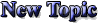Announcement: Be excellent to each other.

 Poster Message
The spitemaster
Level: SmiterRank Points: 352
Registered: 06-09-2005
IP: Logged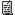Stupid Math formulas (0)
I came accros a funny math equation today. I knew another one that proved studying made you fail but I can't remember it. Well here goes:

girls=time*money
Time=money
therefore girls=money(squared)
(money is the root(Square root) of all evil)
=(square root Evil)(squared)
Girls=Evil

Do you understand now? (Not that good at explaining)

If anyone can show me how to make the equation right (symbols wise) tell me.

____________________________
Last night upon a stair
I met a man that wasn't there
He wasn't there again today
I wish that man would stay away

[Last edited by The spitemaster at 05-02-2006 02:23 AM]
05-02-2006 at 02:14 AM
Someone Else
Level: Smitemaster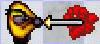Rank Points: 1184
Registered: 06-14-2005
IP: LoggedRe: Stupid Math formulas (0)
3*4=12 therefore
12/4=3

anything*0=0 therefore
0/0=anything therefore
anything=anything therefore
1=2 therefore
Mathematics is the product of a deranged imagination.
05-02-2006 at 02:28 AM
Hix
Level: Master DelverRank Points: 106
Registered: 12-31-2004
IP: LoggedRe: Stupid Math formulas (+2)
Problem 1 -- Prove that a crocodile is longer than it is wide.

We first prove the following:
Lemma -- "A crocodile is longer than it is green"
To see this, just examine the crocodile: It's long on the top and on the bottom, but it's only green on the top. Thus, it is longer than it is green, proving the lemma.
Now note that the crocodile is green along both its length and its width, but it is only wide along its width. Therefore, it is greener than it is wide. This, along with the lemma, completes the proof that a crocodile is longer than it is wide.

Problem 2 -- Compute the sum: 1+2+4+8+16+32+...

Let S = 1+2+4+8+16+32+...

S = 2S - S [by simple algebraic identity]
= (2+4+8+16+32+...) - (1+2+4+8+16+32) [by definition of S]
= -1 [by cancelling like terms]

Thus, 1+2+4+8+16+32+... = -1

Both of these proofs are, of course, flawlessly consistant. I must, however, mention that it is only the love of money that is the root of all evil. I'm not sure what changes this makes to the Girls=Evil proof, though.
05-02-2006 at 04:39 PM
Chris
Level: Master Delver
Rank Points: 144
Registered: 08-12-2005
IP: LoggedRe: Stupid Math formulas (+3)
I think that changes the result, giving:

"the love of square girls is evil"

05-02-2006 at 05:01 PM
Pinnacle
Level: SmitemasterRank Points: 1088
Registered: 06-10-2004
IP: LoggedRe: Stupid Math formulas (0)
Let (right) = 1

Since wrong is the opposite of right,
Let (wrong) = -1

(wrong)(wrong) = (right)
Two wrongs make a right*

*as long as you multiply them.

____________________________
~Ambrose Bierce, The Devil's Dictionary
05-02-2006 at 06:21 PM
stigant
Level: Smitemaster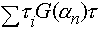Rank Points: 1173
Registered: 08-19-2004
IP: LoggedRe: Stupid Math formulas (+1)
Prove: All serpents have an infinite number of legs.
We will proceed by induction on the size of a group of serpents. Consider a group of 0 serpents. All the serpents in that group have an infinite number of legs. (None of the serpents in the group don't have a infinite number of legs). Now, suppose that in a group of n-1 serpents, all the serpents have an infinite number of legs. Consider a group of n serpents. This can clearly be divided into a group of n-1 serpents and a group of 1 serpents. By our supposition, all of the serpents in the group of n-1 serpents have an infinite number of legs. Now, switch the lone serpent with one of the snakes in the group of n-1. We have a new group of n-1 serpents, and so we see that our lonely serpent must also have an infinite number of legs. Thus, in a group of n serpents, all serpents have an infinite number of legs. Now, the number of serpents in the world is a finite number, N. Consider the group of all serpents. This group must consist only of serpents with an infinite number of legs. Therefore, all serpents have an infinite number of legs.

____________________________
Progress Quest Progress
05-02-2006 at 06:36 PM
jbluestein
Level: SmitemasterRank Points: 1644
Registered: 12-23-2005
IP: LoggedRe: Stupid Math formulas (0)
quote:
stigant wrote:
Prove: All serpents have an infinite number of legs.
We will proceed by induction on the size of a group of serpents. Consider a group of 0 serpents. All the serpents in that group have an infinite number of legs. (None of the serpents in the group don't have a infinite number of legs). Now, suppose that in a group of n-1 serpents, all the serpents have an infinite number of legs. Consider a group of n serpents. This can clearly be divided into a group of n-1 serpents and a group of 1 serpents. By our supposition, all of the serpents in the group of n-1 serpents have an infinite number of legs. Now, switch the lone serpent with one of the snakes in the group of n-1. We have a new group of n-1 serpents, and so we see that our lonely serpent must also have an infinite number of legs. Thus, in a group of n serpents, all serpents have an infinite number of legs. Now, the number of serpents in the world is a finite number, N. Consider the group of all serpents. This group must consist only of serpents with an infinite number of legs. Therefore, all serpents have an infinite number of legs.

An impressively serpentine mental contortion.

____________________________
"Rings and knots of joy and grief, all interlaced and locking." --William Buck
05-02-2006 at 06:41 PM
krammer
Level: SmitemasterRank Points: 902
Registered: 02-12-2003
IP: LoggedRe: Stupid Math formulas (0)
quote:
stigant wrote:
We will proceed by induction on the size of a group of serpents.
Flaw - serpents don't form a group. (They don't tend to be associative as a rule, being solitary creatures.) I think you mean a set of serpents.

____________________________
+++ Divide by Cucumber Error +++ Please Reinstall Universe and Reboot +++

"Oook!"
05-02-2006 at 07:21 PM
stigant
Level: SmitemasterRank Points: 1173
Registered: 08-19-2004
IP: LoggedRe: Stupid Math formulas (0)
Well I suppose we could add a lemma: A set of serpents forms a group... We will proceed by induction on the size of the set....

:-)

____________________________
Progress Quest Progress
05-02-2006 at 07:49 PM
jbluestein
Level: SmitemasterRank Points: 1644
Registered: 12-23-2005
IP: LoggedRe: Stupid Math formulas (+1)
quote:
stigant wrote:
Well I suppose we could add a lemma: A set of serpents forms a group... We will proceed by induction on the size of the set....

:-)

Well, a serpent can certainly form a ring...

____________________________
"Rings and knots of joy and grief, all interlaced and locking." --William Buck
05-02-2006 at 08:33 PM
Kevin_P86
Level: SmitemasterRank Points: 534
Registered: 06-28-2005
IP: LoggedRe: Stupid Math formulas (0)
However, that is certainly not a valid induction. In general, Mathematical Induction works by establishing the following implication chain, where P(n) is the proposition for the value n (in this case, P(n) = "all sets of n serpents consist only of snakes with an infinite number of legs"):

P(1) => P(2) => P(3) => P(4) => ... etc

In order to establish this chain, you need a starting point (in this case, P(1), although it doesn't have to be 1), and the general implication P(k) => P(k+1) for some arbitrary k.

In your case, the flaw in the induction falls when n = 1, and therefore n-1 = 0. When "switch[ing] the lone serpent with one of the snakes in the group of n-1", you assume the set of n-1 snakes is nonempty. If it is empty, there is no other snake in that group from which to infer the lone snake has infinite legs.

Therefore, you have proven the following:

P(0) ^ (P(1) => P(2) => P(3) => ...)

which is not sufficient for an inductive proof, since the falsity of P(1) would nullify the entire chain (which it so happens is the case) since any implication is true if the antecendent is false.

____________________________
+++++[>+++++<-]>[>+++>++++>+++++<<<-]>.>+.>-------.<++++.+++++.
05-02-2006 at 10:12 PM
Kevin_P86
Level: SmitemasterRank Points: 534
Registered: 06-28-2005
IP: LoggedRe: Stupid Math formulas (0)
There's another "proof" I have run across that "proves" 2 = 1:

a = b
a^2 = ab
a^2 - b^2 = ab - b^2
(a - b)(a + b) = b(a - b)
a + b = b
b + b = b
2b = b
2 = 1

------------------------------------------

Another "proof" that "proves" any two numbers are equal:

Let a and b be any two distinct (unequal) numbers.
Let c be their average, so that a + b = 2c.

Multiply both sides of the equation by ab to obtain
a^2  b^2 = 2ac  2bc.

Add b^2  2ac + c^2 to both sides to obtain
a^2 - 2ac + c^2 = b^2  2bc + c^2.

Both sides of the previous equation are now perfect squares and the equation can be written
(a - c)^2 = (b - c)^2.

Take the square root of both sides; then
a  c = b  c

from which it follows that a = b, contrary to our initial assumption.

____________________________
+++++[>+++++<-]>[>+++>++++>+++++<<<-]>.>+.>-------.<++++.+++++.
05-02-2006 at 10:19 PM
Chris
Level: Master Delver
Rank Points: 144
Registered: 08-12-2005
IP: LoggedRe: Stupid Math formulas (0)
quote:
Kevin_P86 wrote:
There's another "proof" I have run across that "proves" 2 = 1:

a = b
a^2 = ab
a^2 - b^2 = ab - b^2
(a - b)(a + b) = b(a - b)
a + b = b
b + b = b
2b = b
2 = 1

Since you found the fault in the induction above, I thought I would point out the fault here... To get from line 4 to line 5 you divide both sides by (a - b), but a = b, so you're dividing by zero. While it's true that 0*2 = 0*1, I can't divide both sides by zero to conclude that 2 = 1.

I'm curious to know what's wrong with the "1 + 2 + 4 + ... = -1" proof though. Anyone?
05-02-2006 at 11:04 PM
stigant
Level: SmitemasterRank Points: 1173
Registered: 08-19-2004
IP: LoggedRe: Stupid Math formulas (0)
The series 1+2+4+8+.... clearly doesn't converge, so subtracting it from both sides or multiplying by 2 etc have no meaning.

____________________________
Progress Quest Progress
05-02-2006 at 11:42 PM
silver
Level: Smitemaster
Rank Points: 913
Registered: 01-18-2005
IP: LoggedRe: Stupid Math formulas (0)
quote:
Chris wrote:
I'm curious to know what's wrong with the "1 + 2 + 4 + ... = -1" proof though. Anyone?

"S = 2S - S [by simple algebraic identity]"

doesn't work with infinities.

____________________________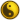05-02-2006 at 11:44 PM
Maurog
Level: Smitemaster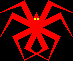Rank Points: 1497
Registered: 09-16-2004
IP: LoggedRe: Stupid Math formulas (0)
Nothing is wrong, except the part when you cancel a positive infinity with a negative infinity. "S + S = 2S" works only on numbers. For infinity you get "S + S = S" because you cannot use basic math functions on it like it was a number.

And of course "taking square root of both sides" is not consistent because square root is not a function - you get two roots for each side.

____________________________
Slay the living! Raise the dead!
Paint the sky in crimson red!

[Last edited by Maurog at 05-02-2006 11:57 PM]
05-02-2006 at 11:56 PM
coppro
Level: Smitemaster
Rank Points: 1306
Registered: 11-24-2005
IP: LoggedRe: Stupid Math formulas (0)
Well, I would say that one could assume that if

A=B
then
sqrt(A)=sqrt(B)

Prove to me an opposite.
05-03-2006 at 12:03 AM
Chris
Level: Master Delver
Rank Points: 144
Registered: 08-12-2005
IP: LoggedRe: Stupid Math formulas (0)
I received an email telling me that Kevin_P86 had posted a reply here, in which he used the word 'injective'. When I came to look, it's not here. How can that be?
05-03-2006 at 01:11 AM
Kevin_P86
Level: SmitemasterRank Points: 534
Registered: 06-28-2005
IP: LoggedRe: Stupid Math formulas (0)
A = B indeed does not imply sqrt(A) = sqrt(B) since, as was mentioned, sqrt is not a function since sqrt(4) = 2 and sqrt(4) = -2 simultaneously, for example.

Therefore, (a - c)^2 = (b - c)^2 does not necessarily imply (a - c) = (b - c). That would only be the case if (a - c) and (b - c) were either both positive or both negative. In this case, from the equation a + b = 2c it follows directly that (a - c) and (b - c) have different signs, specifically (a - c) = (c - b).

____________________________
+++++[>+++++<-]>[>+++>++++>+++++<<<-]>.>+.>-------.<++++.+++++.
05-03-2006 at 01:12 AM
Kevin_P86
Level: SmitemasterRank Points: 534
Registered: 06-28-2005
IP: LoggedRe: Stupid Math formulas (0)
Sorry Chris - I deleted it to reformulate my thoughts...Above is essentially equivalent to my original reply.

____________________________
+++++[>+++++<-]>[>+++>++++>+++++<<<-]>.>+.>-------.<++++.+++++.
05-03-2006 at 01:13 AM
KevG
Level: SmiterRank Points: 333
Registered: 08-16-2004
IP: LoggedRe: Stupid Math formulas (0)
quote:
Kevin_P86 wrote:A = B indeed does not imply sqrt(A) = sqrt(B)
Actually, A = B does imply sqrt(A) = sqrt(B). sqrt(A) is defined to be the positive root. This is a different statement from A^2 = B^2 does not imply A = B.
05-03-2006 at 02:47 AM
silver
Level: Smitemaster
Rank Points: 913
Registered: 01-18-2005
IP: Logged

File: sqrta.bmp (1.8 KB)Re: Stupid Math formulas (0)
quote:
KevG wrote:
quote:
Kevin_P86 wrote:A = B indeed does not imply sqrt(A) = sqrt(B)
Actually, A = B does imply sqrt(A) = sqrt(B). sqrt(A) is defined to be the positive root. This is a different statement from A^2 = B^2 does not imply A = B.

there's a difference between using the java/C function and using the C notation to indicate a mathematical relation. in a math class, sqrt(A) is just the ASCII way of expressing this:which has two solutions (the postive and the negative). square-root is NOT a function, it's "merely" a relation.

However, there's a separate algebraic rule forbidding dividing by unbound variables (because you can't guarantee you're avoiding division by 0), which is the real flaw in the "proof"

____________________________[Last edited by silver at 05-03-2006 07:35 AM]
05-03-2006 at 07:26 AM
KevG
Level: SmiterRank Points: 333
Registered: 08-16-2004
IP: LoggedRe: Stupid Math formulas (0)
quote:
silver wrote:
there's a difference between using the java/C function and using the C notation to indicate a mathematical relation. in a math class, sqrt(A) is just the ASCII way of expressing this:which has two solutions (the postive and the negative). square-root is NOT a function, it's "merely" a relation.
The equation x^2 = 4 has two solutions, the equation x = sqrt(4) only has one. My point, which admittedly was a nitpick, is that in standard mathematical notation the expressiononly refers to the postive root of A. If you wish to refer to both roots you put a plus/minus sign in front. It has nothing to do with the actual properties of the square root operation, it's simply a notational quirk.
05-03-2006 at 09:09 AM
Chris
Level: Master Delver
Rank Points: 144
Registered: 08-12-2005
IP: LoggedRe: Stupid Math formulas (0)
quote:
silver wrote:
However, there's a separate algebraic rule forbidding dividing by unbound variables (because you can't guarantee you're avoiding division by 0), which is the real flaw in the "proof"

But in the proof which involves taking the square root of both sides there was no division, was there? The flaw does seem to be that it's not valid to go from

(a - c)^2 = (b - c)^2.

to

(a - c) = (b - c)

05-03-2006 at 10:30 AM
stigant
Level: SmitemasterRank Points: 1173
Registered: 08-19-2004
IP: LoggedRe: Stupid Math formulas (0)
You are correct, that step is not valid. But the problem is not that you aren't allowed to square-root both sides of an equation.
The problem is in how you simplify the square roots on both sides. Essentially, sqrt(x^2) != x (again, still indicating positive square root). The correct simplification is sqrt(x^2) = |x| (absolute value of x). So taking the square roots of both sides of
(a-b)^2 = (b-c)^2
gives
sqrt((a-b)^2) = sqrt((b-c)^2)
which simplifies to
|a-b| = |b-c|

____________________________
Progress Quest Progress
05-03-2006 at 01:24 PM
Pilchard VIII
Level: SmiterRank Points: 392
Registered: 01-21-2006
IP: LoggedRe: Stupid Math formulas (+1)
I can prove that Barney the dinosaur is the devil!

Take the words CUTE PURPLE DINOSAUR
Change the U's to V's CVTE PVRPLE DINOSAVR
Take out the letters that aren't roman numerals CVVLDIV
add them up... 100 + 5 + 5 + 50 + 500 + 1 +5
What do you get? 666!

____________________________
The DROD Dragon
05-03-2006 at 04:08 PM
silver
Level: Smitemaster
Rank Points: 913
Registered: 01-18-2005
IP: LoggedRe: Stupid Math formulas (0)
quote:
Chris wrote:
quote:
silver wrote:
However, there's a separate algebraic rule forbidding dividing by unbound variables (because you can't guarantee you're avoiding division by 0), which is the real flaw in the "proof"

But in the proof which involves taking the square root of both sides there was no division, was there? The flaw does seem to be that it's not valid to go from

(a - c)^2 = (b - c)^2.

to

(a - c) = (b - c)

whoops, sorry, got confused, I was thinking of these steps:

(a - b)(a + b) = b(a - b)
a + b = b

but that was from a different faux proof.
and I'll concede the sqrt thing, I have been out of the classroom too long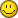____________________________05-03-2006 at 04:40 PM
key-smiter
Level: Master Delver
Rank Points: 121
Registered: 06-15-2003
IP: LoggedRe: Stupid Math formulas (0)
quote:
Pilchard VIII wrote:
I can prove that Barney the dinosaur is the devil!

Take the words CUTE PURPLE DINOSAUR
Change the U's to V's CVTE PVRPLE DINOSAVR
Take out the letters that aren't roman numerals CVVLDIV
add them up... 100 + 5 + 5 + 50 + 500 + 1 +5
What do you get? 666!

Yes, and in the 1980s one could prove that Reagan was the Antichrist . . . his full name was Ronald Wilson Reagan. Each part of his name had six letters, ergo 666.
05-24-2006 at 06:24 PM
key-smiter
Level: Master Delver
Rank Points: 121
Registered: 06-15-2003
IP: LoggedRe: Stupid Math formulas (+1)
Here's my favorite math "formula":

Postulate 1: Knowledge is Power
Postulate 2: Time is Money

Starting with a definition from physics: Power = (Work) / (Time)

Since, from our postulates, Knowledge = Power, and Work = Time, Knowledge = (Work) / (Money)
Or, rearranging, Money = (Work) / (Knowledge).

Thus, as knowledge approaches zero, the money earned approaches infinity regardless of the work actually done. Or, simply phrased, the less you know, the more you make.
05-24-2006 at 06:32 PM
Bunches
Level: Master DelverRank Points: 192
Registered: 03-17-2006
IP: LoggedRe: Stupid Math formulas (+1)
key-smiter, my name has 6 letters in my first, middle and last name. Even Ezlo will tell you that i'm not that evil!
05-24-2006 at 06:52 PM